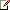# TDistribution Method

Measurement Studio 2012 for Visual Studio 2010 Help

Edition Date: August 2012

Part Number: 372636F-01

»View Product InfoDownload Help (Windows Only)Note: This topic applies to the following Measurement Studio editions: Enterprise.

Calculates probability using a T distribution.

Namespace: NationalInstruments.Analysis.Math

Assembly: NationalInstruments.Analysis.Enterprise (in NationalInstruments.Analysis.Enterprise.dll) Version: 12.0.40.318

## Syntax

Visual Basic (Declaration)
```<SecurityPermissionAttribute(SecurityAction.Demand, Flags := SecurityPermissionFlag.UnmanagedCode)> _
Public Shared Function TDistribution ( _
x As Double, _
degreesOfFreedom As Integer _
) As Double```

C#
```[SecurityPermissionAttribute(SecurityAction.Demand, Flags = SecurityPermissionFlag.UnmanagedCode)]
public static double TDistribution(
double x,
int degreesOfFreedom
)```

### Parameters

x
Type: System.Double
The point to which the probability is calculated.
degreesOfFreedom
Type: System.Int32
Degrees of freedom of the distribution.

### Return Value

The computed probability.

## Exceptions

ExceptionCondition
ArgumentException degreesOfFreedom is less than or equal to zero.
DllNotFoundException The analysis library cannot be found.
EntryPointNotFoundException A required operation in the analysis library cannot be found.

## Remarks

Computes the one-sided probability, p, of the t-distributed random variable, Tn, with the specified degreesOfFreedom

p = Prob{Tn x}

where T is t-distributed,
p is the probability,
n is degreesOfFreedom, and
x is the value.

## Examples

The following example uses the TDistribution static method.
VB.NET
```Dim x, p As Double
Dim degreesOfFreedom As Integer

x = 1.258
degreesOfFreedom = 6

p = Probability.TDistribution(x, degreesOfFreedom)```
C#
```double x, p;
int degreesOfFreedom;

x = 1.258;
degreesOfFreedom = 6;

p = Probability.TDistribution(x, degreesOfFreedom);```